Calculates and returns an overlay of contour lines for the current height map.

generate_contour_overlay(
heightmap,
levels = NA,
nlevels = NA,
zscale = 1,
width = NA,
height = NA,
color = "black",
linewidth = 1
)

## Arguments

heightmap A two-dimensional matrix, where each entry in the matrix is the elevation at that point. All grid points are assumed to be evenly spaced. Default NA. Automatically generated with 10 levels. Default NA. Controls the auto-generation of levels. If levels is length-2, this will automatically generate nlevels breaks between levels and levels. Default 1. The ratio between the x and y spacing (which are assumed to be equal) and the z axis. For example, if the elevation levels are in units of 1 meter and the grid values are separated by 10 meters, zscale would be 10. Default NA. Width of the resulting overlay. Default the same dimensions as heightmap. Default NA. Width of the resulting overlay. Default the same dimensions as heightmap. Default black. Color. Default 1. Line width.

## Value

Semi-transparent overlay with contours.

## Examples

#Add contours to the montereybay dataset

# \donttest{
montereybay %>%
plot_map()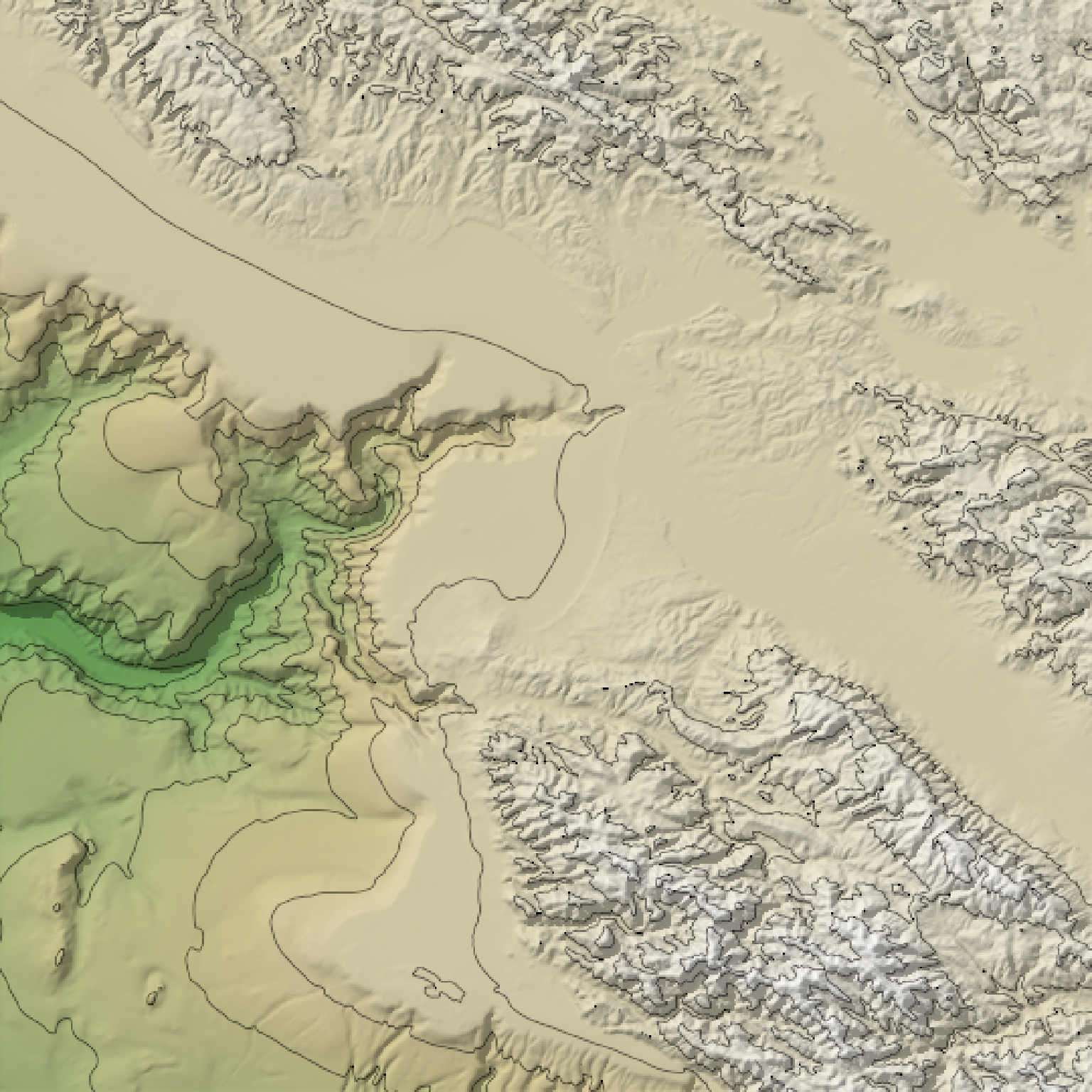#Add a different contour color for above and below water, and specify levels manually
water_palette = colorRampPalette(c("darkblue", "dodgerblue", "lightblue"))(200)
bathy_hs = height_shade(montereybay, texture = water_palette)
breaks = seq(range(montereybay),range(montereybay),length.out=50)
water_breaks = breaks[breaks < 0]
land_breaks = breaks[breaks > 0]

montereybay %>%
add_overlay(generate_contour_overlay(montereybay, levels = water_breaks, color="white"))  %>%
add_overlay(generate_contour_overlay(montereybay, levels = land_breaks, color="black"))  %>%
plot_map()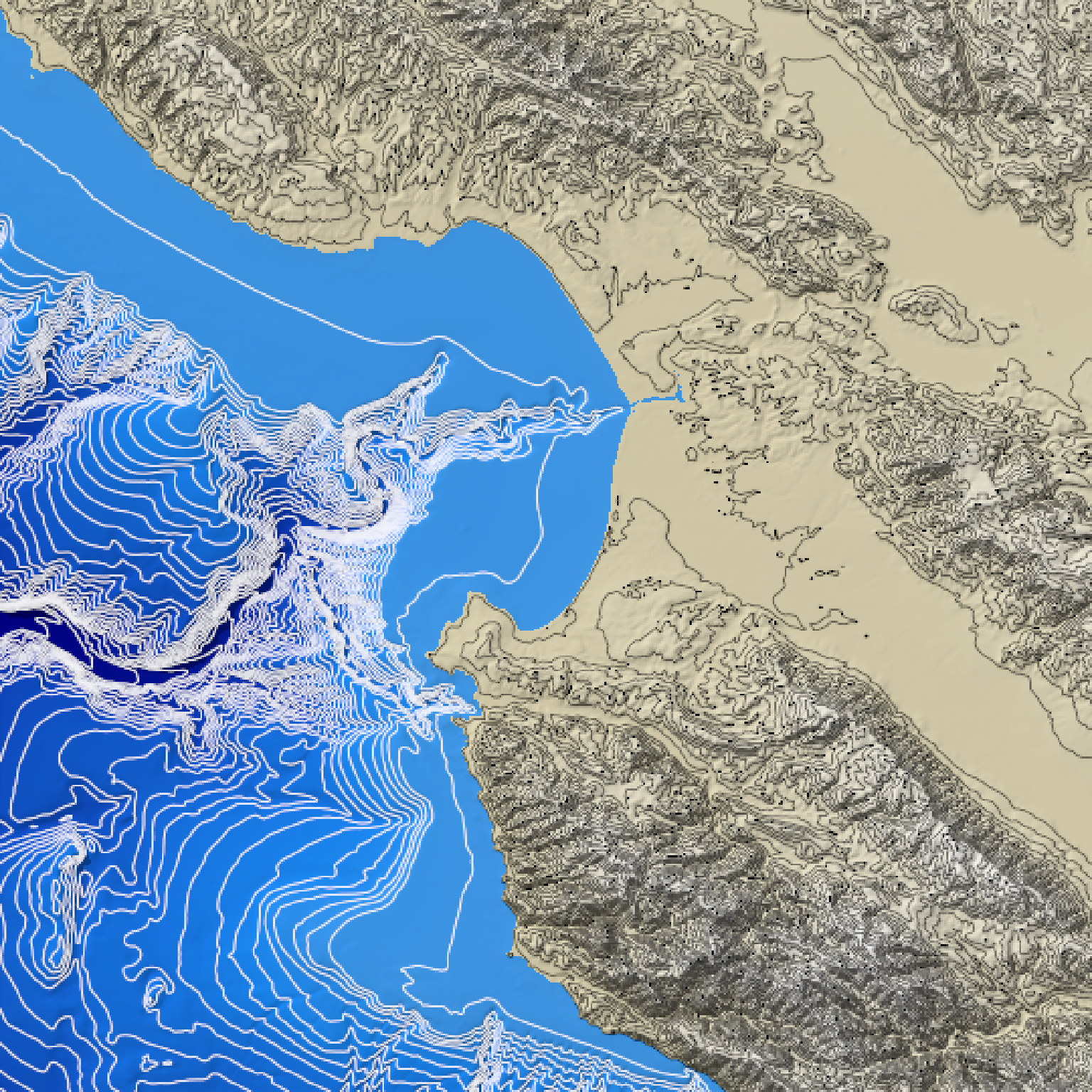#Increase the resolution of the contour to improve the appearance of lines
montereybay %>%
height = nrow(montereybay)*2,
width  = ncol(montereybay)*2))  %>%
height = nrow(montereybay)*2,
width  = ncol(montereybay)*2))  %>%
plot_map()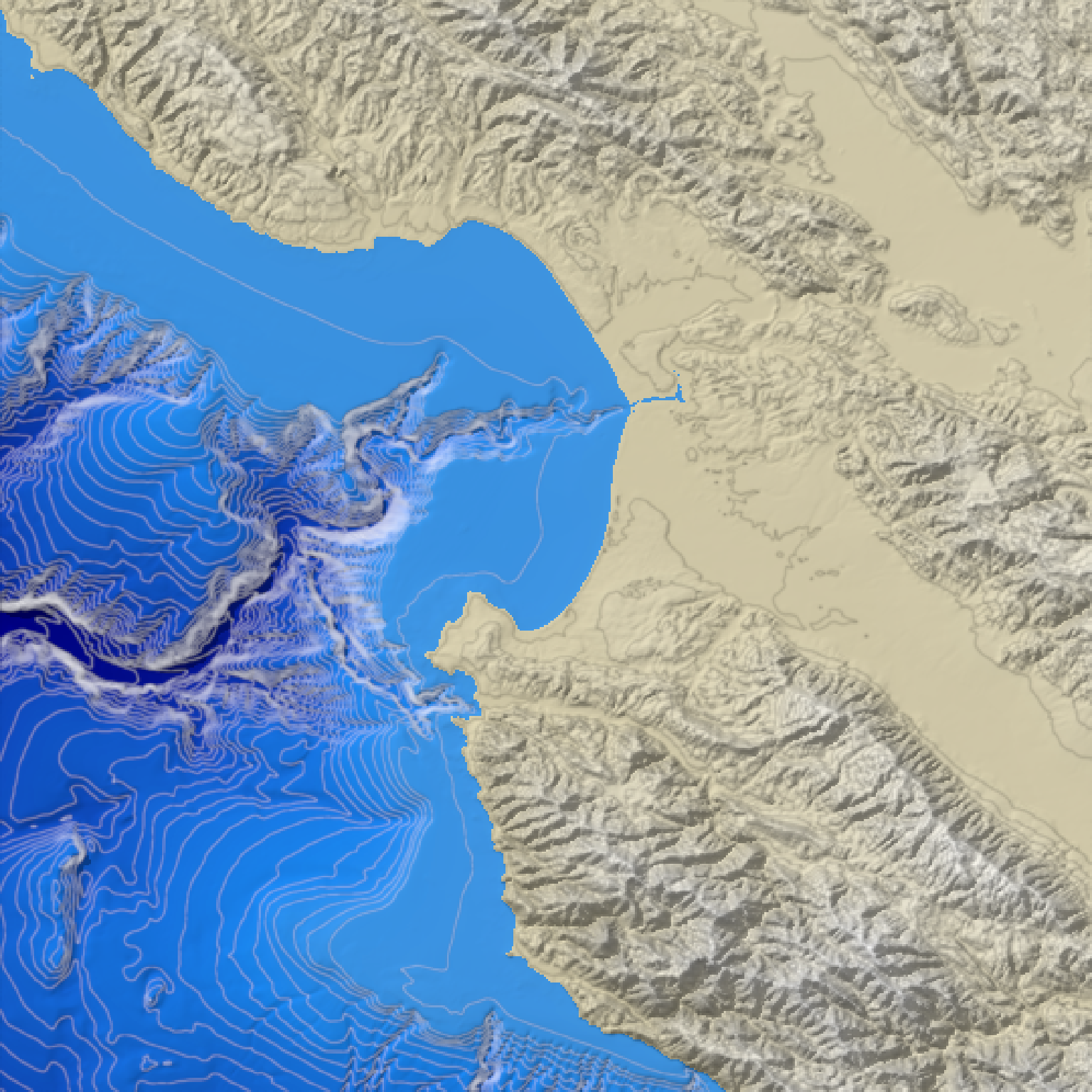#Increase the number of breaks and the transparency (via add_overlay)
montereybay %>%
plot_map()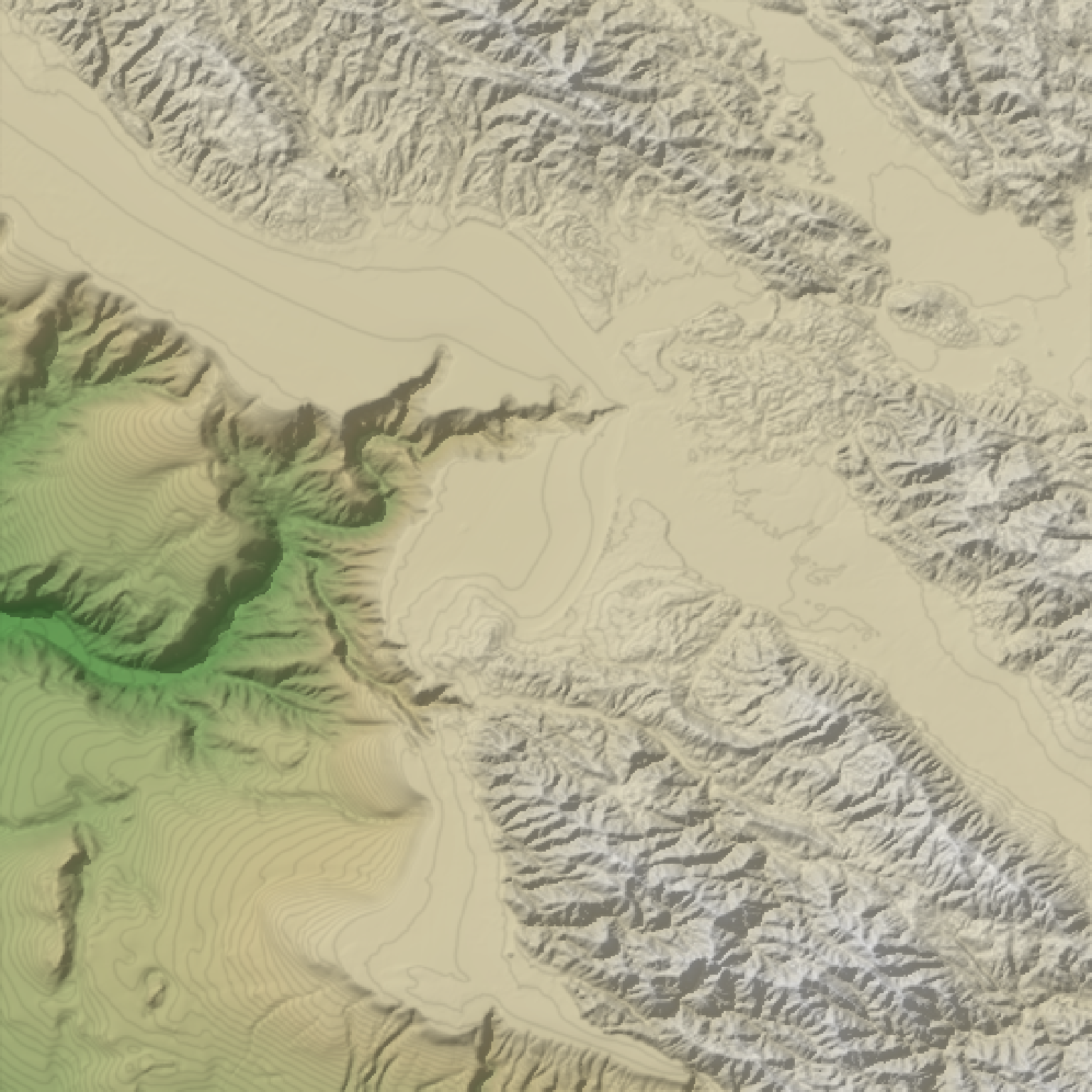plot_map()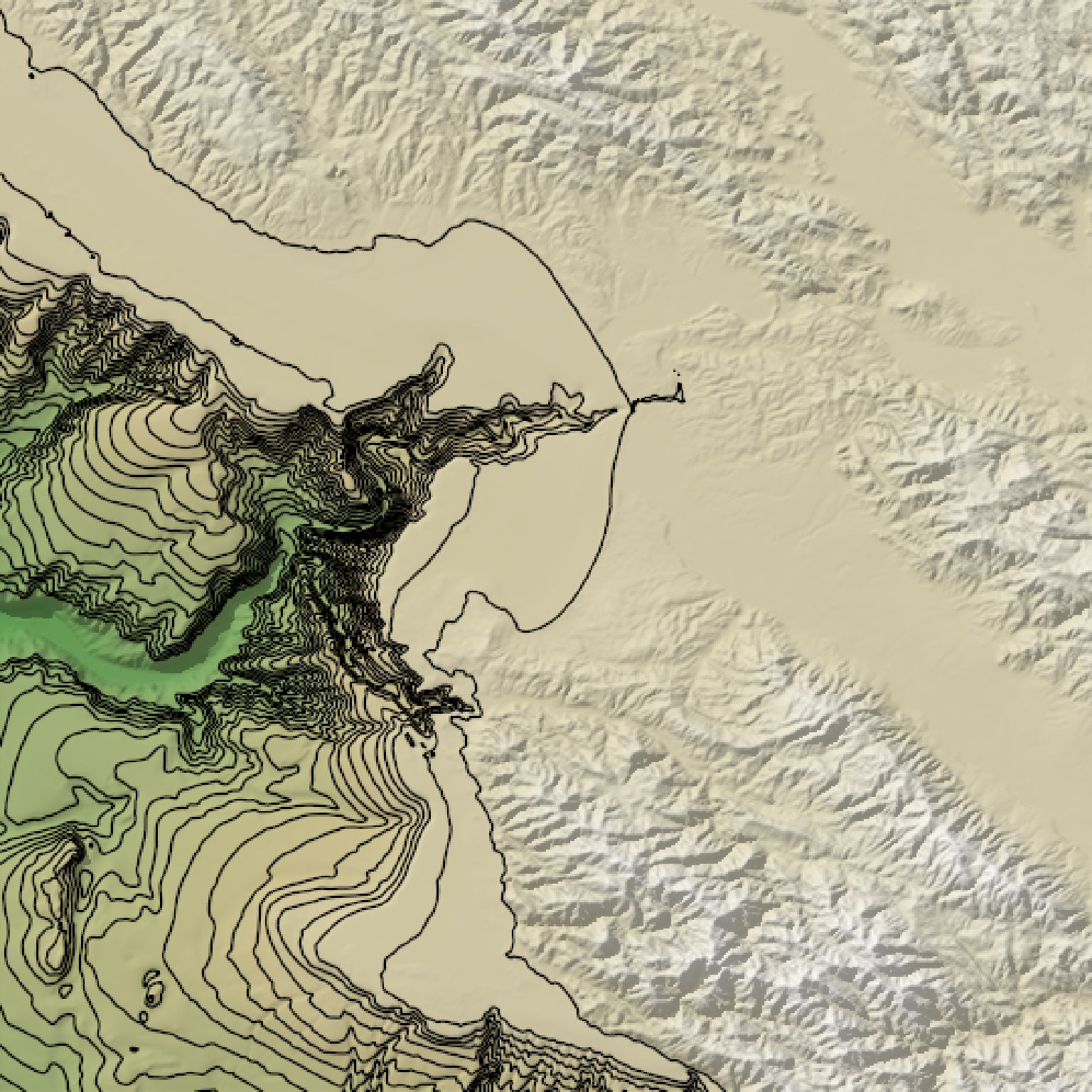# }